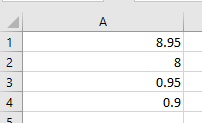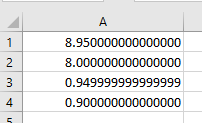Excel / Floating point rounding

Ran into an interesting issue with Excel’s handling of floating point numbers.

Take the following formula:

= IF((8.95-8) = .95, TRUE, FALSE)

You’d think that would return TRUE, but it does not.

If I enter 8.95 into cell A1, and 8 into cell A2, the formula =A2-A1 into cell A3, and =Round(A3,1) into cell A4, I get:Shouldn’t 0.95 round up to 1? Cell A4 show 0.9.

If I change the format of those cells to Number (15 decimal places), it shows that 8.95 - 8 is not exactly 0.95This became apparent when processing timeclock data. The timeclock system must have similar issues. Time is reported in hours, rounding to 10ths. From 8:00 to 8:57 is 57 minutes, which is exactly 0.95 hr, which should round up to 1.0 hours. But the timeclock system shows that as 0.9 hrs - like it was actual 0.9499999999 , which rounds to 0.9.

When comparing floats in older, er, other languages, I got used to writing this type of expression for equality:

abs(numOne - numTwo) < 0.001

You can vary the number based on the precision you want. Probably would work in Excel too.

This is interesting. I can duplicate your problem. I have not seen this before, but I would be interested in hearing about any solutions that come up.

In Excel if you want to round to a whole number you enter 0 for the number of digits.

=Round(A3,0)

I found that adding the rounding in here can fix your if statement:

=IF(ROUND((8.95-8),2)=0.95,TRUE,FALSE)

check you the Precision as Displayed option in Excel:

Thanks for posting!
Nate

1 Like

In my days of embedded programming on 8 bit processors, I would do something similar. I first ran across floating point imprecision when calculating statistics. To my surprise,
6 * 19.9 <> 19.9 + 19.9 + 19.9 + 19.9 + 19.9 + 19.9

edit

It was even worse than the values not being equal. I was trying to take the square root of a difference (which should never be able to be less than zero), and it turned out that
6 * 19.9 - (19.9 + 19.9 + 19.9 + 19.9 + 19.9 + 19.9) was less than zero, and threw a NEG SQRT ERR, when it should have been the square root of zero.

1 Like

A1 - A2 = 0.95 ???
The Excel issue is the subtraction